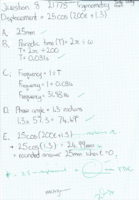# Piston displacement

#### Flimjabs

##### New member
Hi everybody
Displacment =25cos(200t+1.3)
I'm stuck on part E which is
Where does the piston sit when t=0
I have attached the making to this thread, can someone check my reworking, I'm assuming I need to convert radians to degrees so
25cos(1.3×57.3)=6.68mm is this the position when t=0 or do I subtract that from 25mm?

#### Attachments

• 980.8 KB Views: 4

#### HallsofIvy

##### Elite Member
I simply cannot read what you have- it is too small.

#### Dr.Peterson

##### Elite Member
Displacment =25cos(200t+1.3)
I'm stuck on part E which is
Where does the piston sit when t=0
I have attached the making to this thread, can someone check my reworking, I'm assuming I need to convert radians to degrees so
25cos(1.3×57.3)=6.68mm is this the position when t=0 or do I subtract that from 25mm?
Here is an enlarged copy:The note in blue, which I can still barely read, says "radians". That means, "set your calculator to radian mode before you calculate this."

Or, as you suggest, you could manually convert to radians, and you get 6.687 for the expression. (Be sure to round correctly!)

Why would you subtract from 25? I see the blue note at the bottom says so, but since you haven't shown us the actual problem, I have no way to know. If the expression is said to give the displacement, and you were asked for the displacement, then you don't need to do more than you said.

There is a reason we ask you to show the entire problem: